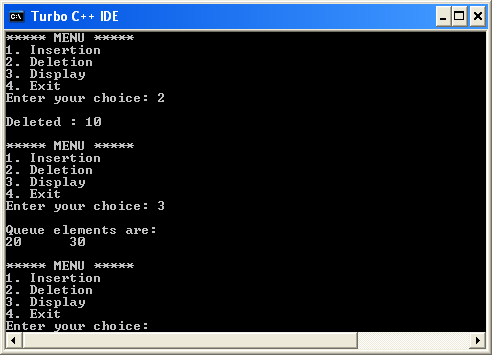The perfect place for easy learning...

×

# Queue Datastructure Using Array

A queue data structure can be implemented using one dimensional array. The queue implemented using array stores only fixed number of data values. The implementation of queue data structure using array is very simple. Just define a one dimensional array of specific size and insert or delete the values into that array by using FIFO (First In First Out) principle with the help of variables 'front' and 'rear'. Initially both 'front' and 'rear' are set to -1. Whenever, we want to insert a new value into the queue, increment 'rear' value by one and then insert at that position. Whenever we want to delete a value from the queue, then delete the element which is at 'front' position and increment 'front' value by one.

# Queue Operations using Array

Queue data structure using array can be implemented as follows...

Before we implement actual operations, first follow the below steps to create an empty queue.

• Step 1 - Include all the header files which are used in the program and define a constant 'SIZE' with specific value.
• Step 2 - Declare all the user defined functions which are used in queue implementation.
• Step 3 - Create a one dimensional array with above defined SIZE (int queue[SIZE])
• Step 4 - Define two integer variables 'front' and 'rear' and initialize both with '-1'. (int front = -1, rear = -1)
• Step 5 - Then implement main method by displaying menu of operations list and make suitable function calls to perform operation selected by the user on queue.

# enQueue(value) - Inserting value into the queue

In a queue data structure, enQueue() is a function used to insert a new element into the queue. In a queue, the new element is always inserted at rear position. The enQueue() function takes one integer value as a parameter and inserts that value into the queue. We can use the following steps to insert an element into the queue...

• Step 1 - Check whether queue is FULL. (rear == SIZE-1)
• Step 2 - If it is FULL, then display "Queue is FULL!!! Insertion is not possible!!!" and terminate the function.
• Step 3 - If it is NOT FULL, then increment rear value by one (rear++) and set queue[rear] = value.

# deQueue() - Deleting a value from the Queue

In a queue data structure, deQueue() is a function used to delete an element from the queue. In a queue, the element is always deleted from front position. The deQueue() function does not take any value as parameter. We can use the following steps to delete an element from the queue...

• Step 1 - Check whether queue is EMPTY. (front == rear)
• Step 2 - If it is EMPTY, then display "Queue is EMPTY!!! Deletion is not possible!!!" and terminate the function.
• Step 3 - If it is NOT EMPTY, then increment the front value by one (front ++). Then display queue[front] as deleted element. Then check whether both front and rear are equal (front == rear), if it TRUE, then set both front and rear to '-1' (front = rear = -1).

# display() - Displays the elements of a Queue

We can use the following steps to display the elements of a queue...

• Step 1 - Check whether queue is EMPTY. (front == rear)
• Step 2 - If it is EMPTY, then display "Queue is EMPTY!!!" and terminate the function.
• Step 3 - If it is NOT EMPTY, then define an integer variable 'i' and set 'i = front+1'.
• Step 4 - Display 'queue[i]' value and increment 'i' value by one (i++). Repeat the same until 'i' value reaches to rear (i <= rear)

# Implementation of Queue Datastructure using Array - C Programming

``````#include<stdio.h>
#include<conio.h>
#define SIZE 10

void enQueue(int);
void deQueue();
void display();

int queue[SIZE], front = -1, rear = -1;

void main()
{
int value, choice;
clrscr();
while(1){
printf("1. Insertion\n2. Deletion\n3. Display\n4. Exit");
scanf("%d",&choice);
switch(choice){
case 1: printf("Enter the value to be insert: ");
scanf("%d",&value);
enQueue(value);
break;
case 2: deQueue();
break;
case 3: display();
break;
case 4: exit(0);
default: printf("\nWrong selection!!! Try again!!!");
}
}
}
void enQueue(int value){
if(rear == SIZE-1)
printf("\nQueue is Full!!! Insertion is not possible!!!");
else{
if(front == -1)
front = 0;
rear++;
queue[rear] = value;
printf("\nInsertion success!!!");
}
}
void deQueue(){
if(front == rear)
printf("\nQueue is Empty!!! Deletion is not possible!!!");
else{
printf("\nDeleted : %d", queue[front]);
front++;
if(front == rear)
front = rear = -1;
}
}
void display(){
if(rear == -1)
printf("\nQueue is Empty!!!");
else{
int i;
printf("\nQueue elements are:\n");
for(i=front; i<=rear; i++)
printf("%d\t",queue[i]);
}
}```
```

Output